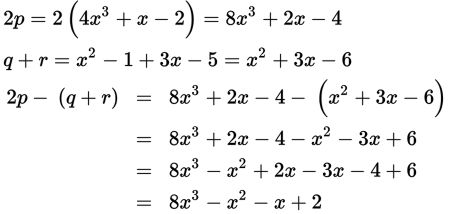# SAT Math Multiple Choice Question 246: Answer and Explanation

### Test Information

Question: 246

6. If p = 4x3 + x - 2, q = x2 - 1, and r = 3x - 5, then what is 2p - (q + r)?

• A. 7x3 - x + 2
• B. 8x3 - x2 - x + 2
• C. 8x3 - x2 - x - 10
• D. 8x3 - x2 + 5x - 8

Explanation:

B

Difficulty: Medium

Category: Passport to Advanced Math / Exponents

Strategic Advice: Attention to detail is the key to answering this question correctly. You simply need to combine like terms, being careful to distribute negative signs where appropriate.

Getting to the Answer: Break the calculation into steps: Find 2p, find q + r, and then subtract the results. Arranging the terms in descending order will help keep them organized.